# Properties of Air: A Manual for Use in Biophysical Ecology, 5th Edition

#### 2019-10-08

Current Addresses:
C. Richard Tracy
Department of Biology MS-315
University of Nevada, Reno
Reno, NV 89557, USA

William R. Welch
Deceased; Madison, Wisconsin, USA

Berry Pinshow
Mitrani Department of Desert Ecology
Jacob Blaustein Institutes for Desert Research
Ben-Gurion University of the Negev
Sede Boqer Campus
84990 Midreshet Ben-Gurion, Israel

Michael R. Kearney
School of BioSciences
The University of Melbourne
Victoria, 3010 Australia

Warren P. Porter
Department of Zoology
University of Wisconsin-Madison
Zoology Research Building
1117 West Johnson Street
Madison, WI 53706, USA

## Preface to the Fifth Edition

This fifth edition of Properties of Air has been transcribed into R Markdown, and the Fortran subroutines and function have been converted to R functions, by Michael Kearney. The figures have been generated with the R functions. It is now a vignette as part of the NicheMapR R package.

## Preface to the Fourth Edition

This fourth edition of Properties of Air is largely a reprint of the third edition with errors corrected. This edition was created because Berry Pinshow wanted a digital version for his personal use. He scanned the third edition, and corrected errors in it. The fourth edition exists due to Berry’s enthusiasm to develop a digital version with errors corrected. This preface is largely to say a few things about this small book.

When I was a graduate student, I found myself constantly looking up physical characteristics of air as I developed biophysical ecological models. I got so tired of looking in reference books that I decided to extract what I needed and make tables and graphs of the properties of air. These, and other tables that I accumulated, became my main source of reference material in my research. For years, I carried these reference tools around in a folder of hand-drawn graphs and tables. Various visitors to Warren Porter’s lab asked for copies of the graphs and tables in my folder. Bill Welch suggested that we get the artistic staff in the Department of Zoology at the University of Wisconsin to make pen-and-ink drawings from my graphs. Subsequently, I created a set of general subroutines in the program language FORTRAN, which simply translated the graphs. As these tools accumulated, Bill Welch and I continued to add to the reference material, and Warren Porter facilitated getting the book put together in a package that we self-published out of the Porter Lab. Many dozens of people have requested this book, and it seems to remain useful as many continue to ask for copies of it. However, the original printing has now exhausted, so creating this digital version made sense.

One of the original authors of this book, and our good friend, Dr. Bill Welch, died at much too young an age. To him, we dedicate this fourth edition.

C. Richard Tracy
Department of Biology
University of Nevada, Reno
Reno, NV 89557

First Edition 1973
Second Edition 1978
Third Edition 1980
Fourth Edition 2010

## Preface to the Third Edition

This manual was made possible by the invaluable assistance of Ann Chambers and Cheryle Hughes. Financial support was provided by grants from the Department of Zoology, Wisconsin Alumni Research Foundation, ERDA (Contract EY-76-5-02-2270), and NSF (Grant Nos. 74-19454 and 77-25786 to WPP).

First edition 1973
Second edition 1978
Third edition 1980
Fourth edition 2010

## Introduction

This manual comprises a series of tables and graphs that illustrate selected properties of air as functions of temperature, pressure, and humidity. The particular properties that are displayed were chosen because of their importance in analytical studies of energy (heat) and mass (water) transfer between organisms and their physical environments. Except where otherwise noted, the graphs were drawn from information in the Smithsonian Meteorological Tables (List 1971). These graphs are considered to be a concise references for the values of the indicated properties, and not substitutes for the more accurate Smithsonian tables.

All information in this manual is expressed in terms of the International System of Units (SI), which is based, in part, on the metre, kilogram, second and kelvin. Basic units, derived units, prefixes, and some useful physical constants and conversion factors are listed below. More extensive lists are provided by List (1971), Mechtly (1973), and the Symbols Committee of the Royal Society (1975). Note that the symbol for a physical quantity is an italicized (slanted) letter of the Roman or Greek alphabet, and the unit for a quantity is indicated by an unitalicized (upright) Roman letter.

An equation is provided as a mathematical description of each graph. In addition, two Fortran subroutines and a function that calculate all of the information in the graphs are included in the NicheMapR package as R functions, and the R code use to obtain the data in the plots is provided as code snippets below each figure. Two of the equations in function DRYAIR (thermal conductivity of air and latent heat of vaporization of water) are linear regressions that were fit to tabular data. All other equations in DRYAIR were taken from the Smithsonian tables.

All humidity calculations in function WETAIR are made with the internationally accepted Goff-Gratch equation (function VAPPRS used by function WETAIR). A mathematically less cumbersome and often more useful alternative was reported by Tetens (Murray 1976); calculations with this equation differ negligibly from those with the Goff-Gratch expression. Teten’s equation is provided in Figure 4 to calculate the saturation vapor pressure ($$e_d^*$$) at the dewpoint temperature ($$t_d$$). Note that this equation can also be used to calculate the saturation vapor pressure ($$e^*$$) at the dry bulb temperature ($$t$$) if $$t$$ replaces $$t_d$$, and the saturation vapor pressure ($$e_w^*$$) at the wet bulb temperature ($$t_w$$) if $$t_w$$ replaces $$t_d$$. The relationship of wet bulb temperature to dry bulb temperature, vapor density, and relative humidity is shown in the psychometric diagram Figure 15.

Mathematical expressions for some properties of air that are dependent on humidity are not available. The equations for these properties are restricted to dry air in this manual but see Mason and Monchick (1965) for further information on humid air.

## Table 1. Basic units

Physical Quantity Quantity Symbol Unit Unit Symbol
length l metre m
mass m kilogram kg
time t second s
thermodynamic temperature T kelvin K

## Table 2. Derived units

Physical Quantity Quantity Symbol Unit Unit Symbol Unit Definition
force F newton N $$J$$ $$m^{-1}$$
energy E joule J $$N$$ $$m$$
pressure p pascal Pa $$N$$ $$m^{-2}$$, $$J$$ $$m^{-3}$$
power P watt W $$J$$ $$s^{-1}$$
volume t cubic metre $$m^3$$ $$m^3$$
Celsius temperature$$^*$$ $$t$$ degree Celsius °C $$K$$

## Table 3. Prefixes

Multiple Prefix Symbol Multiple Prefix Symbol
$$10^{-1}$$ deci d $$10^{1}$$ deca da
$$10^{-2}$$ centi c $$10^{2}$$ hecto h
$$10^{-3}$$ milli m $$10^{3}$$ kilo k
$$10^{-6}$$ micro $$\mu$$ $$10^{6}$$ mega M
$$10^{-9}$$ nano n $$10^{9}$$ giga G
$$10^{-12}$$ pico p $$10^{12}$$ tera T

## Table 4. Conversion factors

Force
dyne = $$10^5$$ N$$^*$$
kilogram force = $$9.80665$$ N$$^*$$
ounce force = $$2.7801385$$ x $$10^{-1}$$ N
pound force = $$4.4482216152605$$ N$$^*$$

Energy
British thermal unit (thermochemical) = $$1.054350$$ x $$10^{3}$$ J
calorie (thermochemical) = $$4.184$$ J
what hour = $$3.60$$ x $$10^{3}$$ J$$^*$$

Pressure
bar = $$10^{5}$$ $$Pa^*$$
centimetre of water (4 °C) = $$9.80638$$ x $$10^{1}$$ Pa
millimetre of mercury (0 °C) = $$1.333224$$ x $$10^{2}$$ Pa
pound per square inch = $$6.894757$$ x $$10^{3}$$ Pa
standard atmosphere = $$1.01325$$ x $$10^{5}$$ Pa
torr = $$1.333224$$ x $$10^{2}$$ Pa

Power
British thermal unit (thermochemical) = $$1.054350$$ x $$10^{3}$$ W
calorie (thermochemical) per second = $$4.184$$ W$$^*$$
calorie (thermochemical) per minute = $$6.973333$$ x $$10^{-2}$$ W
kilocalorie (thermochemical) per hour = $$8.604207$$ x $$10^{-1}$$ W

Volume
cubic foot = $$2.8316592$$ m$$^{3*}$$
gallon = $$3.785411784$$ x $$10^{-3}$$ m$$^{-3*}$$
litre = $$10^{-3}$$ m$$^{-3*}$$
pint (U.S. liquid) = $$4.73176473$$ x $$10^{-4}$$ m$$^{3*}$$
quart (U.S. liquid) = $$9.4635295$$ x $$10^{-4}$$ m$$^{3*}$$

Length
foot = $$3.048$$ x $$10^{-1}$$ m$$^{*}$$
inch = $$2.54$$ x $$10^{-2}$$ m$$^{*}$$
mile (U.S. statute) = $$1.609344$$ x $$10^{3}$$ m$$^{*}$$
mile (U.S. nautical) = $$1.852$$ x $$10^{3}$$ m$$^{*}$$
yard = $$9.144$$ x $$10^{-1}$$ m$$^{*}$$

Mass     ounce = $$2.8349523125$$ x $$10^{-2}$$ kg$$^{*}$$
pound = $$4.5359237$$ x $$10^{-1}$$ kg$$^{*}$$
ton (short, 2000 pound) = $$9.0718474$$ x $$10^{2}$$ kg$$^{*}$$

$$^{*}$$exact, by definition

## Table 5. Physical constants

Quantity Symbol Value Units
Density of dry air at 25 °C and 101325 Pa$$^{*}$$ $$\rho$$ $$1.184$$ kg m$$^3$$
Diffusivity of water vapor in air at 25 °C and 101325 Pa$$^{*}$$ D $$2.614$$ x $$10^{-5}$$ m$$^2$$ s$$^{-1}$$
Dynamic viscosity of dry air at 25 °C and 101325 Pa$$^{*}$$ $$\mu$$ $$1.806$$ x $$10^{-5}$$ kg m$$^{-1}$$ s$$^{-1}$$
Gas constant for ideal gas R $$8.31434$$ J mol$$^{-1}$$ K$$^{-1}$$
Gas constant for water vapor R$$_v$$ $$4.6150$$ x $$10^{2}$$ Pa m$$^3$$ kg$$^{-1}$$ K$$^{-1}$$
Gravitational constant R $$6.6732$$ x $$10^{-11}$$ N m$$^2$$ kg$$^{-2}$$
Kinematic viscosity of dry air at 25 °C and 101325 Pa$$^{*}$$ $$\nu$$ $$1.525$$ x $$10^{-5}$$ m$$^3$$ s$$^{-1}$$
Latent heat of vaporization of water at 25 °C L $$2.442$$ x $$10^{6}$$ J kg$$^{-1}$$
Molecular weight of dry air M$$_a$$ $$2.8966$$ x $$10^{-2}$$ kg mol$$^{-1}$$
Molecular weight of water M$$_v$$ $$1.8016$$ x $$10^{-2}$$ kg mol$$^{-1}$$
Specific heat of dry air c$$_p$$ $$1.00484$$ x $$10^{3}$$ K kg$$^{-1}$$ K$$^{-1}$$
Standard pressure P$$_0$$ $$1.01325$$ x $$10^{5}$$ Pa
Standard temperature T$$_0$$ $$2.7315$$ x $$10^{2}$$ K
Stefan-Boltzmann constant $$\sigma$$ $$5.67032$$ x $$10^{-8}$$ W m$$^{-2}$$ K$$^{-4}$$
Thermal conductivity of dry air at 25 °C and 101325 Pa$$^{*}$$ k $$2.601$$ x $$10^{-2}$$ W m$$^{-1}$$ K$$^{-1}$$

$$^{*}$$Variable that is functionally related to other properties in this manual

## Table 6. Percent relative humidity over saturated solutions as a function of temperature. From Winston and Bates (1960)$$^1$$

Compound 10°C 15°C 20°C 25°C 30°C 35°C
NH$$_4$$Cl 79.0 79.5 79.5 78.0 77.5 -
NH$$_4$$HPO$$_4$$ (monophosphate) - - 93.0 93.0 92.0 -
NH$$_4$$NO$$_3$$ 75.0 70.0 65.5 62.5 59.5 55.0
NH$$_4$$NO$$_3$$ + NaNO$$_3$$ 58.0 55.0 52.0 50.0 47.0 44.5
NH$$_4$$NO$$_3$$ + AgNO$$_3$$ 70.5 68.0 65.1 61.5 58.0 55.0
(NH$$_4$$)$$_2$$ SO$$_4$$ 80.5 81.0 80.5 80.0 80.0 79.5
CaCl$$_2$$·6H$$_2$$O$$^2$$ 38.0 35.0 32.5 29.5 - -
CaHPO$$_4$$·2H$$_2$$O$$^3$$ 97.5 99.5 95.0 97.0 95.0 -
CaH$$_4$$(PO$$_4$$)·H$$_2$$O$$^4$$ 98.0 99.0 94.0 96.0 93.5 -
Ca(NO$$_3$$)$$_2$$·4H$$_2$$O$$^5$$ - 56.0 55.5 50.5 47.0 -
C0Cl$$_2$$$$^6$$ - 72.5 (18 °C) 67.0 - 62.0 -
Cr$$_2$$O$$_3$$$$^7$$ - 45.5 (39.0) - 44.5 -
Glucose 57 (12 °C) - 55.0 55.0 - 53.0
LiCl·H$$_2$$O$$^8$$ 13.5 13.0 12.5 12.0 11.5 11.5
Pb(NO$$_3$$)$$_2$$$$^9$$ 98.0 97.0 97.0 95.5 95.0 94.5
Pb(NO$$_3$$)$$_2$$+NH$$_4$$NO$$_3$$ 66.5 62.0 58.0 55.0 52.5 49.5
MgCl$$_2$$·6H$$_2$$O 34.0 34.0 33.0 32.5 32.5 32.5
Mg(NO$$_3$$)$$_2$$·6H$$_2$$O 58.0 56.0 55.0 53.0 52.0 50.5
P$$_2$$O$$_5$$ 0.0 0.0 0.0 0.0 0.0 -
KAc (21.0) - 20.0 22.5 22.0 -
KBr 86.0 - 84.0 80.0 82.0 -
K$$_2$$CO$$_3$$·2H$$_2$$O$$^{10}$$ 47.0 44.0 44.0 43.0 43.0 -
KCl 88.0 86.5 85.0 85.0 84.5 83.0
K$$_2$$CrO$$_4$$ - - 88.0 - 86.5 -
K$$_2$$Cr$$_2$$O$$_7$$ - - 98.0 98.0 97.5 96.5
KH$$_2$$PO$$_4$$ 98.0 99.0 96.5 96.0 93.5 -
KF·2H$$_2$$O$$^{11}$$ - - - 30.5 27.4 -
KNO$$_3$$$$^{12}$$ 96.0 95.5 93.5 92.5 91.0 89.5
KNO$$_2$$ - - 48.5 - 47.0 -
K$$_2$$SO$$_4$$ 98.5 99.0 98.0 97.5 96.5 96.0
KCNS$$^{13}$$ 52.0 50.0 47.0 46.5 43.5 41.5
K tartrate$$^{14}$$ - 75.0 75.0 75.0 74.0 -
KNa tartrate$$^{15}$$ 87.5 - 87.0 87.0 87.0 -
Pyrocatechol - 99.0 95.5 93.5 92.5 90.5
Resorinol - 95.0 87.0 85.0 82.5 79.5
AgNO$$_3$$ 88.0 86.0 84.0 82.0 80.0 78.0
AgNO$$_3$$+Pb(NO$$_3$$)$$_2$$ 84.0 83.0 80.5 78.5 76.5 75.0
NaAc·3H$$_2$$O - - 75.0 73.0 71.0 -
NaBr·2H$$_2$$O$$^{16}$$ 63.0 61.0 59.0 57.5 56.0 54.5
Na$$_2$$CO$$_3$$·10H$$_2$$O$$^{17}$$ (99.0) - 92.0 87.0 87.0 -
NaCl 76.5 76.0 76.0 75.5 75.5 75.5
NaCl+KCl - - 70.0 71.5 71.0 -
Na$$_2$$CrO$$_4$$·4H$$_2$$O - - - - 64.5 -
Na$$_2$$Cr$$_2$$O$$_7$$·H$$_2$$O$$^{18}$$ 60.0 56.5 54.5 53.0 52.5 51.0
NaOH (5.5) - 5.5 7.0 (4.0) -
NaI - - 38.0 38.0 36.0 -
NaNO$$_3$$ 77.5 76.5 76.0 74.0 72.5 71.0
NaNO$$_2$$ - - 65.5 64.0 63.0 -
Na$$_2$$SO$$_4$$·10H$$_2$$O - - (93.0) (93.0) - (87.0)
Na tartrate$$^{19}$$ - 94.0 92.0 92.0 92.0 -
Urea 81.5 80.0 80.0 76.0 73.3 -
ZnCl$$_2$$·1.5H$$_2$$O$$^{20}$$ (10.0) (10.0) 10.0 - (10.0) -
ZnSO$$_4$$·7H$$_2$$O$$^{21}$$ - - - 88.5 - -

$$^{1}$$Parentheses around a value of relative humidity indicate some doubt of accuracy.
$$^{2}$$May be unstable around 20 °C.
$$^{3}$$May be a transition point between 15 and 20 °C.
$$^{4}$$Calcium monophosphate.
$$^{5}$$Good up to 40 °C.
$$^{6}$$Uncertain around 20 °C, large temperature coefficient.
$$^{7}$$Other researchers report 40% at 30 °C.
$$^{8}$$LiCl·XH$$_2$$O → LiCl·H$$_2$$O at 25 °C.
$$^{9}$$Solution slightly acid due to hydrolysis, but stable.
$$^{10}$$Should be granulated then moistened; powdered from is unstable.
$$^{11}$$KF·2H$$_2$$O → KF at 50 °C.
$$^{12}$$Large temperature coefficient.
$$^{13}$$Very toxic.
$$^{14}$$Potassium tartrate. Excellent.
$$^{15}$$Potassium sodium tartrate (Rochelle salts). Good only below 40 °C.
$$^{16}$$Good up to 55 °C, large temperature coefficient.
$$^{17}$$NaCO$$_3$$·10H$$_2$$O…..>Na$$_2$$CO$$_3$$·H$$_2$$O at 32 °C.
$$^{18}$$Very good; small temperature coefficient.
$$^{19}$$This is also listed with one water of hydration, instead of two; good only below 40 °C.
$$^{20}$$Unstable around 20 °C.
$$^{21}$$Good below 10 °C.

## Table 7. Percent relative humidity over saturated solutions at 20 °C. From Winston and Bates (1960)

Compound Relative Humidity Compound Relative Humidity
BaI$$_2$$·2H$$_2$$O 44.0 NaHSO$$_4$$ 52.0
CaSO$$_4$$·2H$$_2$$O 98.0 NaBrO$$_3$$ 92.0
NH$$_4$$·4H$$_2$$O 65.0 NaNO$$_3$$+KNO$$_3$$ 71.0
NH$$_4$$·7H$$_2$$O 90.0 Na$$_2$$HPO$$_4$$·7H$$_2$$O 95.0
NH$$_4$$·4H$$_2$$O 57.5 Na$$_2$$SO$$_3$$$$^*$$ 95.0
NH$$_4$$·6H$$_2$$O 55.5 NaCNS 35.5
H$$_3$$PO$$_4$$ 9.0 Na$$_2$$S$$_2$$O$$_3$$ 78.0
KHSO$$_4$$ 86.0 2n(NO$$_3$$)$$_2$$ 42.0
K$$_2$$HPO$$_4$$ 44.5 - -

$$^{*}$$Good only below 29.6 °C.

## Table 8. Percent relative humidity over saturated solutions at 25 °C. From Winston and Bates (1960)$$^1$$

Compound Relative Humidity Compound Relative Humidity
NH$$_4$$Br$$_2$$ 75.0 MgSO$$_4$$·7H$$_2$$O 89.0
BaBr$$_2$$ 74.5 Mg(CNS)$$_2$$ 47.5
BaCl$$_2$$ 90.0 MnBr$$_2$$·6H$$_2$$O 34.5
BaI$$_2$$·2H$$_2$$O 43.0 MnCl$$_2$$·4H$$_2$$O 56.0
Ba(CNS)$$_2$$·2H$$_2$$O 54.5 NiBr$$_2$$·3H$$_2$$O 27.0
CaAc$$_2$$·H$$_2$$O 17.0 NiCl$$_2$$·6H$$_2$$O 53.0
CaAc$$_2$$·H$$_2$$O + sucrose 13.0 H$$_3$$PO$$_4$$ 9.0
CaBr$$_2$$ 16.5 KBr + sucrose 63.0
CaI$$_2$$·6H$$_2$$O 11.5 KBr + Urea 51.0
Ca methane sulphonate 72.5 KClO$$_3$$ 98.0
Ca(MnO$$_4$$)$$_2$$·4H$$_2$$O 37.5 KCl + KClO$$_3$$ 85.0
Ca(CNS)$$_2$$·3H$$_2$$O 17.5 KHCO$$_4$$ 21.5
CaZnCl$$_2$$ 20.0 KOH 8.0
CeCl$$_3$$ (tech.) 45.5 K$$_4$$P$$_2$$O$$_7$$·3H$$_2$$O 49.5
CrCl$$_3$$ 42.5 K$$_2$$S$$_2$$O$$_3$$ 66.0
CoBr$$_2$$ 41.5 NaAc + sucrose 37.6
Co(NO$$_3$$)$$_2$$·6H$$_2$$O (49.0) NaCl + sucrose 63.0
CuBr$$_2$$ 62.5 NaCl + NaNO$$_3$$ 69.0
CuCl$$_2$$·2H$$_2$$O 67.0 NaCl + Na$$_2$$SO$$_4$$·7H$$_2$$O 74.0
Cu(NO$$_3$$)$$_2$$·6H$$_2$$O (35.0) NaHSO$$_4$$ 81.0
Ethanolamine sulphate 12.5 NaH$$_2$$PO$$_4$$ 70.0
FeBr$$_2$$·6H$$_2$$O 39.0 Na methane sulphonate 61.5
FeCl$$_2$$·4H$$_2$$O 60.0 SrBr$$_2$$·6H$$_2$$O 58.5
LiBr·2H$$_2$$O 7.0 SrI$$_2$$·6H$$_2$$O 33.0
LiI·3H$$_2$$O 18.0 Sr(CNS)$$_2$$·3H$$_2$$O 31.5
LiNo$$_3$$·3H$$_2$$O 47.0 Sucrose 85.0
LiCNS$$^2$$ 12.5 Surcose + urea 52.0
MgBr$$_2$$·6H$$_2$$O 31.5 ZnBr$$_2$$ 8.5
MgI$$_2$$ 27.0 ZbI$$_2$$$$^2$$ 20.0
Mg(ClO$$_4$$)$$_2$$·6H$$_2$$O (41.0) Zn(MnO$$_4$$)$$_2$$·6H$$_2$$O 51.0
Mg silicoflouride 91.5 Zn(CNS)$$_2$$ 80.5

$$^{1}$$Parentheses around a value of relative humidity indicate some doubt of accuracy.
$$^{2}$$Light sensitive.
$$^{3}$$Prepare by mixing Ca(OH)$$_2$$ + ZnO + 4HCl.

## Table 9. Efficiency of drying agents expressed as mass of water remaining per unit volume of air dried at 25 °C, and as relative humidity$$^*$$. From Winston and Bates (1960).

Compound Mass, g m$$^{-3}$$ (STPD) Relative Humidity
P$$_4$$O$$_5$$ <2 x 10$$^{-3}$$ <0.1
Mg(ClO$$_4$$)$$_2$$ <5 x 10$$^{-4}$$ <0.1
Mg(ClO$$_4$$)$$_2$$·3H$$_2$$O <2 x 10$$^{-5}$$ <0.1
KOH (fused) 0.002 <0.1
Al$$_2$$O$$_3$$ 0.003 <0.1
H$$_2$$SO$$_4$$ 0.003 <0.1
MgO 0.008 <0.1
NaOH (fused) 0.16 0.6
CaBr$$_2$$ 0.2 0.8
CaO 0.2 0.8
CaCl$$_2$$ 0.14-0.25 0.6-1.0
H$$_2$$SO$$_4$$ (95%) 0.3 1.2
CaCl$$_2$$ (fused) 0.36 1.4
ZnCl$$_2$$ 0.8 3.2
ZnBr$$_2$$ 1.1 4.4
CuSO$$_4$$ 1.4 5.6

$$^{*}$$Relative humidity calculated as 3.98 x mass.

## Table 10. Water potential (kPa) of NaCl solutions as a function of temperature. From Brown and van Haveren (1972).

#### Temperature, °C

Molality 0 5 10 15 20 25 30 35 40
0.05 -214 -218 -222 -226 -230 -234 -238 -242 -245
0.10 -423 -431 -439 -447 -454 -462 -470 -477 -485
0.20 -8360 -852 -868 -884 -900 -915 -930 -946 -961
0.30 -1247 -1272 -1297 -1321 -1344 -1368 -1391 -1415 -1437
0.40 -1658 -1693 -1727 -1759 -1791 -1823 -1855 -1886 -1917
0.50 -2070 -2115 -2158 -2200 -2241 -2281 -2322 -2362 -2402
0.60 -2484 -2539 -2593 -2644 -2694 -2744 -2794 -2843 -2891
0.70 -2901 -2967 -3030 -3091 -3151 -3210 -3270 -3228 -3385
0.80 -3320 -3398 -3472 -3543 -3612 -3682 -3751 -3818 -3885
0.90 -3743 -3832 -3917 -3998 -4079 -4158 -4327 -4314 -4390
1.00 -4169 -4270 -4366 -4459 -4550 -4640 -4729 -4815 -4901
1.10 -4599 -4713 -4820 -4924 -5026 -5127 -5226 -5322 -5418
1.20 -5032 -5160 -5278 -5394 -5507 -5620 -5730 -5835 -5941
1.30 -5470 -5611 -5742 -5869 -5994 -6119 -6239 -6354 -6471
1.40 -5912 -6068 -6210 -6350 -6487 -6623 -6754 -6880 -7006
1.50 -6359 -6529 -6684 -6837 -6987 -7134 -7276 -7411 -7548
1.60 -6811 -6996 -7163 -7330 -7491 -7652 -7805 -7950 -8007
1.70 -7260 -7460 -7640 -7820 -8000 -8170 -8300 -8490 -8650
1.80 -7730 -7940 -8130 -8330 -8520 -8700 -8880 -8940 -9210
1.90 -8190 -8430 -8630 -8840 -9040 -9240 -9430 -9600 -9780
2.00 -8670 -8920 -9130 -9360 -9570 -9780 -9980 -10160 -10350

## Table 11. Water potential (kPa) of KCl solutions as a function of temperature. From Brown and van Haveren (1972).

#### Temperature, °C

Molality 0 5 10 15 20 25 30 35 40
0.05 -214 -218 -222 -225 -229 -233 -237 -241 -245
0.10 -412 -429 -436 -444 -452 -459 -467 -474 -482
0.15 -625 -637 -648 -660 -671 -683 -694 -705 -716
0.20 -827 -843 -849 -874 -890 -905 -920 -935 -949
0.25 -1029 -1049 -1068 -1087 -1107 -1126 -1145 -1164 -1182
0.30 -1229 -1253 -1277 -1300 -1324 -1347 -1370 -1392 -1414
0.35 -1429 -1457 -1485 -1512 -1540 -1568 -1594 -1620 -1646
0.40 -1628 -1661 -1693 -1724 -1757 -1788 -1819 -1849 -1879
0.45 -1826 -1864 -1901 -1936 -1973 -2009 -2043 -2077 -2111
0.50 -2025 -2067 -2108 -2148 -2190 -2230 -2268 -2306 -2344
0.55 -2222 -2270 -2316 -2360 -2400 -2451 -2493 -2536 -2578
0.60 -2420 -2473 -2523 -2572 -2623 -2672 -2719 -2765 -2811
0.65 -2617 -2675 -2731 -2784 -2840 -2894 -2945 -2995 -3046
0.70 -2814 -2878 -2938 -2996 -3057 -3116 -3171 -3226 -3280
0.75 -3012 -3080 -3146 -3208 -3274 -3338 -3398 -3457 -3516
0.80 -3208 -3283 -3353 -3421 -3292 -3561 -3625 -3688 -3752
0.85 -3405 -3485 -3561 -3633 -3710 -3784 -3852 -3920 -3988
0.90 -3601 -3687 -3769 -3846 -3928 -4007 -4080 -4153 -4225
0.95 -3797 -3889 -3972 -4059 -4147 -4231 -4309 -4386 -4463
1.00 -3993 -4092 -4185 -4272 -4366 -4455 -4538 -4620 -2702

## Table 12. Preparation of standard molal solutions of NaCl and KCl from 2 molal stock solutions. From Brown and van Haveren (1972).

#### Mass of NaCl Solution, g          Mass of KCl Solution, g

Molality Total 2 Molal Stock Water Total 2 Molal Stock Water
0.05 1002.922 27.9221 975 1003.728 28.7278 975
0.10 1005.844 55.8443 950 1007.456 57.4555 950
0.20 1011.689 111.6886 900 1014.911 114.9110 900
0.30 1017.533 167.5328 850 1022.366 172.3665 850
0.40 1023.377 223.3771 800 1029.822 229.8220 800
0.50 1029.221 279.2214 750 1037.277 287.2775 750
0.60 1035.066 335.0657 700 1044.733 344.7330 700
0.70 1040.910 390.9099 650 1052.188 402.1885 650
0.80 1046.754 446.7542 600 1059.644 459.6440 600
0.90 1052.599 502.5985 550 1067.100 517.0995 550
1.00 1058.443 558.4428 500 1074.555 574.5550 500
1.50 1087.664 837.6642 250 1111.832 861.8325 250
2.00 1116.886 1116.8853 0 1149.110 1149.1100 0

## Figure 1. Black-body emittance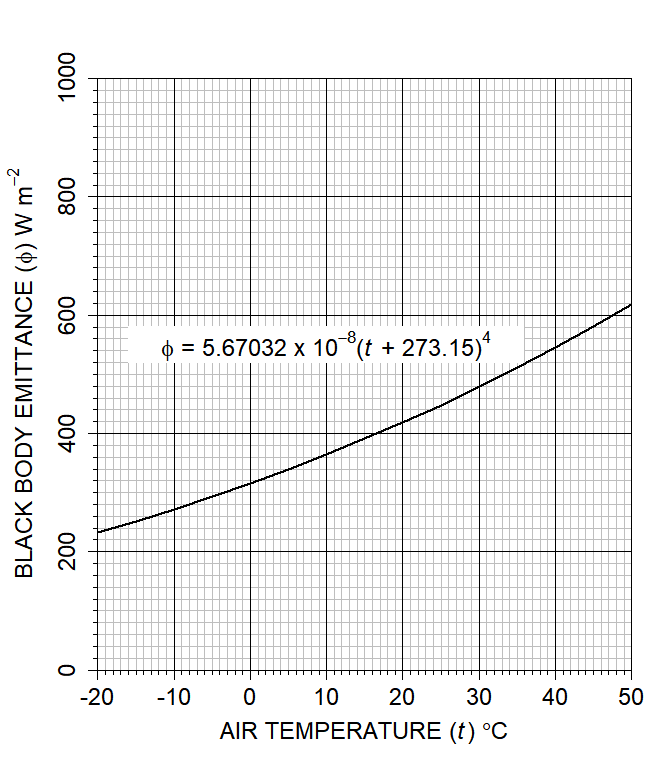tairs=seq(-20,50,5) # sequence of air temperatures

## Figure 3. Dew point temperature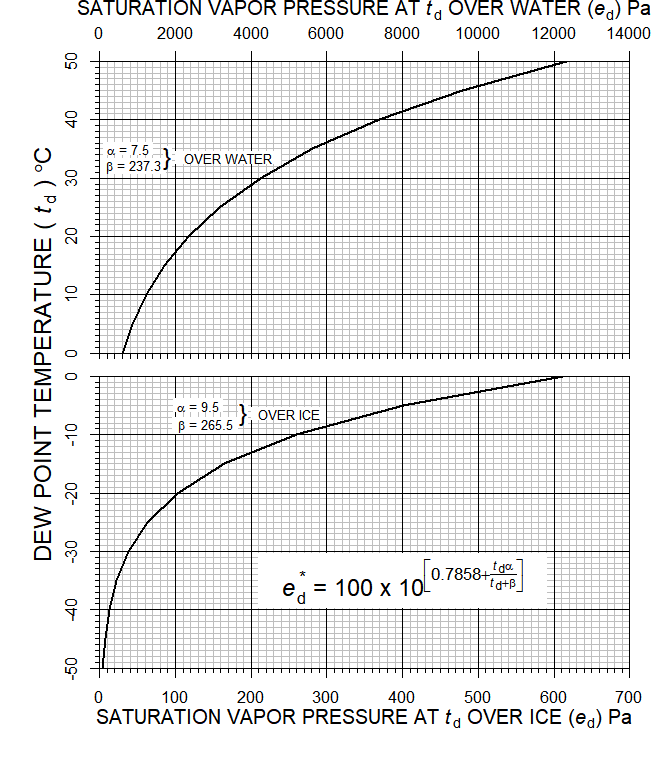dps=seq(-20,50,5) # sequence of dew points

## Figure 5. Dynamic viscosity of air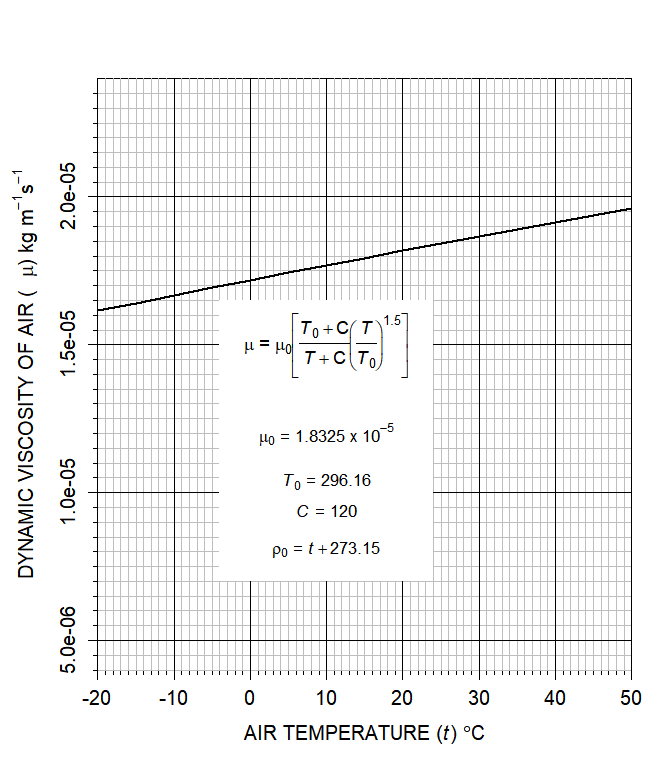tairs=seq(-20,50,5) # sequence of air temperatures

## Figure 7.Kinematic viscosity of air at 100 000 Pa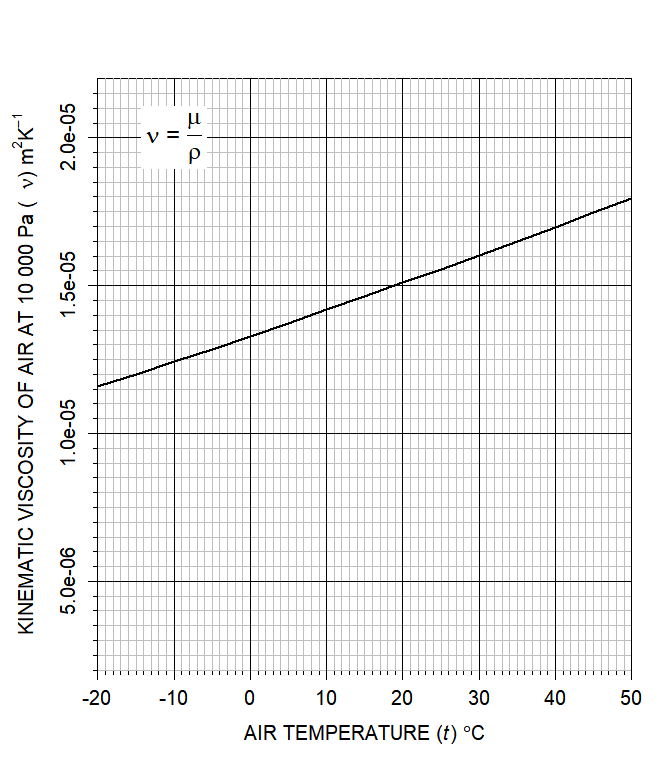tairs=seq(-20,50,5) # sequence of air temperatures

## Figure 9. Mixing ratio over water at 100 000 Pa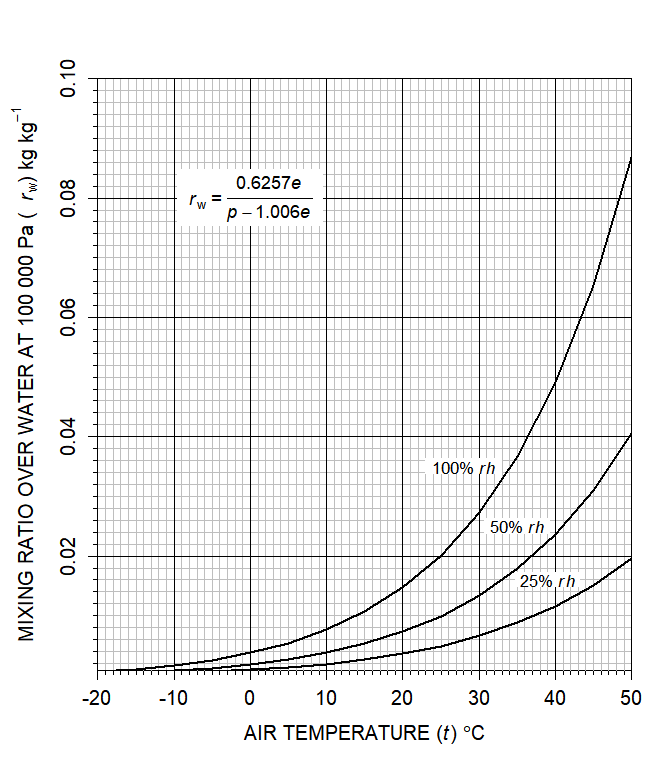tairs=seq(-20,50,5) # sequence of air temperatures

## Figure 11. Standard atmospheric pressure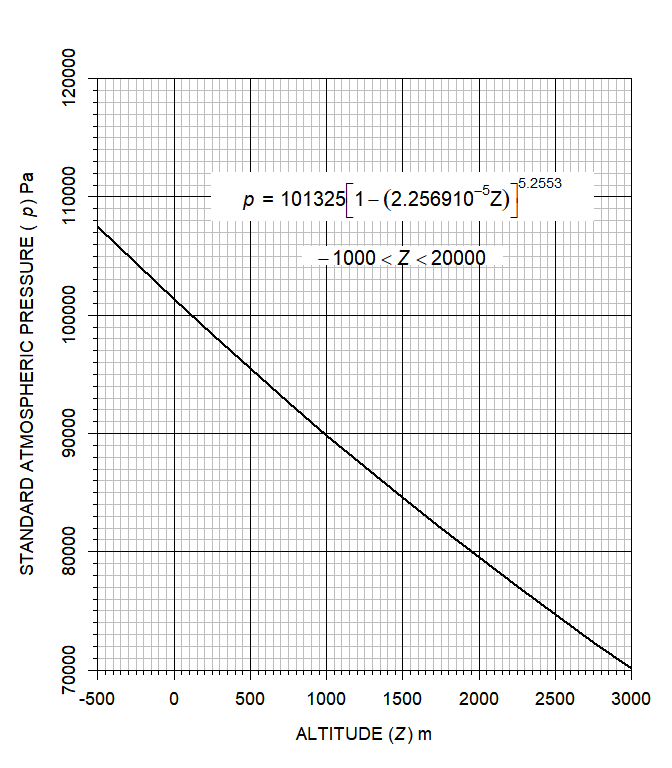alts=seq(-500,3000,250) # sequence of altitudes (m)

## Figure 14. Thermal conductivity of air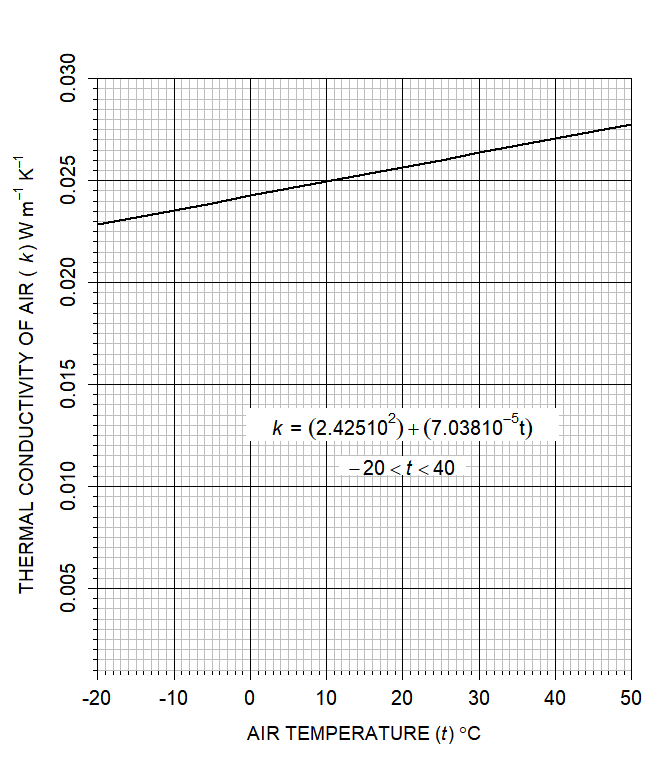tairs=seq(-20,50,5) # sequence of air temperatures
thcond=DRYAIR(db=tairs)$thcond # compute values ## Figure 15. Vapour density over water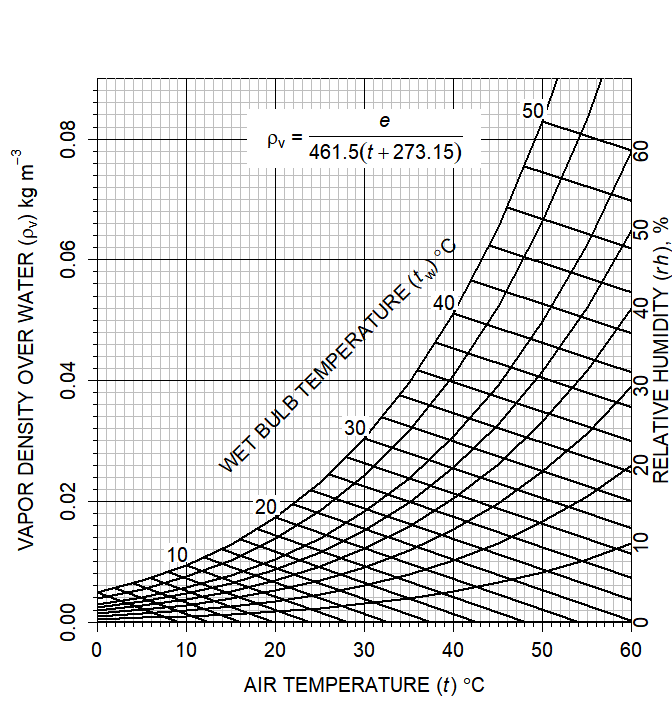# get vapor density at different air temperatures for a given relative humidity tairs=seq(0,60,5) # sequence of air temperatures at which to obtain vapor density vd=WETAIR(db=tairs, rh=100)$vd # obtain vapor density at 100% rh

# get dry bulb temperatures for a set of saturated wet bulb temperatures
wb=seq(0,50,2) # sequence of wet bulb temperatures
# rearrangement of formulae in WETAIR

## Figure 17. Virtual temperature increment at 100 000 Pa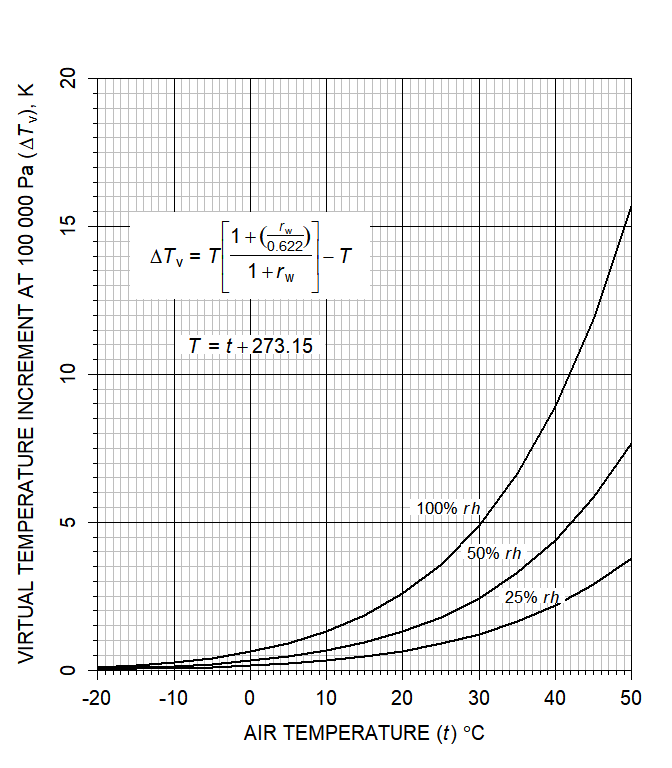tairs=seq(-20,50,5) # sequence of air temperatures

## Figure 19. Wavelength of maximum emittance from black-body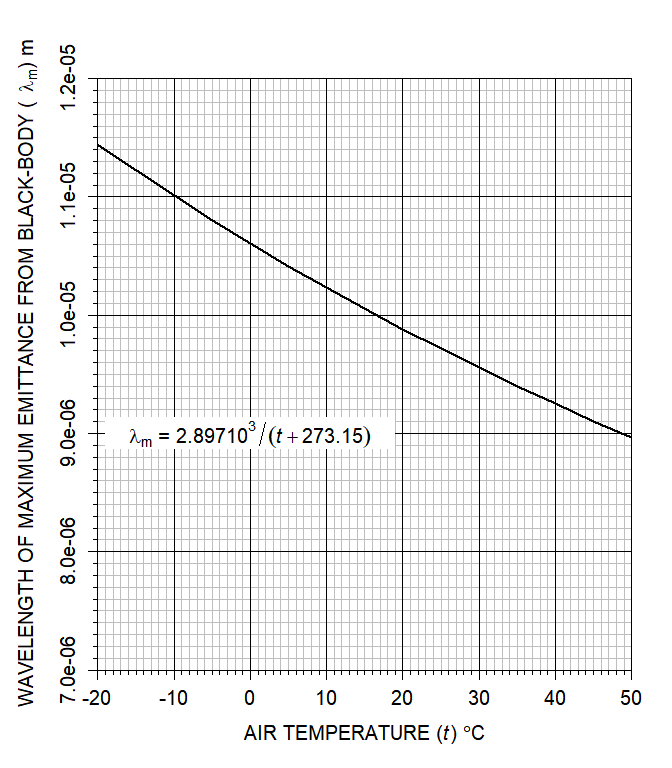tairs=seq(-20,50,5) # sequence of air temperatures
emtmax=DRYAIR(db=tairs)$emtmax # compute values ## Example application on a raster dataset The functions described below can be applied on gridded dataset (rasters). The generic function WETAIR is described below but will not work on rasters (because of conditional statements). Instead, use WETAIR.dp, WETAIR.rh or WETAIR.wb depending on whether you have data on dew point, relative humidity or wet bulb temperature, respectively. The example below is based on code from the function build.global.climate, and adjusts relative humidity for a change in temperature, assuming the absolute humidity (vapor density) remains constant. In the global_climate dataset, this is the assumption made about absolute humidity, with the min and max relative humidity simply based on the max and min air temperatures, respectively. In the calculations below, the maximum humidity for January is calculated by obtaining the vapor pressure for the minimum relative humidity, at the maximum air temperature, then obtaining the saturation vapor pressure at the minimum air temperature and computing the relative humidity at the minimum temperature from the ratio of actual to saturation vapor pressure. The final calculated value should be identical to the maximum relative humidity surface in the global_climate dataset. library(NicheMapR) # load the NicheMapR package library(raster) # package for working with rasters library(ncdf4) # package for dealing with netcdf files (a kind of layered raster) # read the global_climate dataset global_climate<-brick(paste("c:/globalclimate/global_climate.nc",sep="")) # 38th layer of global_climate is min January air temperature*10 Tair_min_january=global_climate[]/10 # 50th layer of global_climate is max January air temperature*10 Tair_max_january=global_climate[]/10 # 62nd layer of global_climate is max January relative humidity*10 RH_min_january=global_climate[]/10 # 74th layer of global_climate is max January relative humidity*10 RH_max_january=global_climate[]/10 # use WETAIR.rh to get the vapor pressure for January based on min # relative humidty and max air temperature e=WETAIR.rh(rh=RH_min_january,db=Tair_max_january)$e
# use the VAPPRS function to get the saturation vapor pressure for
# the new temperature, Tmin January
esat=VAPPRS(Tair_min_january)
# compute new relative humidity for minimum air temperature
RH_max_january=(e/esat)*100
# conditional replace of any values >100 with 100
values(RH_max_january) <- ifelse(values(RH_max_january > 100), 100, values(RH_max_january))
# now plot the results
par(mfrow = c(3,2)) # set up for 6 plots in 2 columns
# plot the January max air temperature
plot(Tair_max_january,zlim=c(-40,50),main="Max Air Temperature, January")
# plot the January min relative humidity
plot(RH_min_january,zlim=c(0,100),main="Min Relative Humidity, January")
plot(e, main="vapor pressure, January")
plot(esat, main="sat. vapor pressure at Tmin")
# plot the January max air temperature
plot(Tair_min_january,zlim=c(-40,50),main="Min Air Temperature, January")
# plot the January min relative humidity
plot(RH_max_january,zlim=c(0,100),main="Max Relative Humidity, January")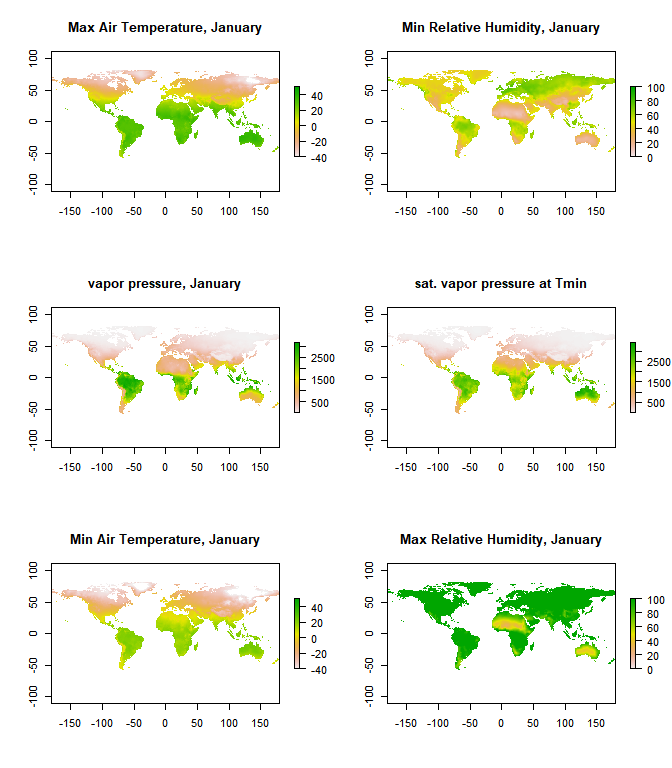## Function DRYAIR

#' DRYAIR
#'
#' Calculates several properties of dry air and related characteristics shown
#' as output variables below. The program is based on equations from List, R. J. 1971.
#' Smithsonian Meteorological Tables. Smithsonian Institution Press. Washington, DC.
#' WETAIR must be used in conjunction with function VAPPRS.
#'
#' The user must supply values for the input variables (db, bp and alt).
#' If alt is known (-1000 < alt < 20000) but not BP, then set BP=0
#' @param db Dry bulb temperature (degrees C)
#' @param bp Barometric pressure (pascal)
#' @param alt Altitude (m)
#' @return patmos Standard atmospheric pressure (P)
#' @return densty Density (kg m-3)
#' @return visdyn Dynamic viscosity (kg m-1 s-1)
#' @return viskin Kinematic viscosity (m2 s-1)
#' @return difvpr Diffusivity of water vapour in air (m2 s-1)
#' @return thcond Thermal conductivity (W m-1 K-1)
#' @return htovpr Latent heat of vapourisation of water (J kg-1)
#' @return tcoeff Temperature coefficient of volume expansion (K-1)
#' @return ggroup Group of variables in Grashof number (1-m3 -K)
#' @return bbemit black body emittance (W m-2)
#' @return emtmax Wave length of maximum emittance (m)
#' @export
DRYAIR <- function(db=db, bp=0, alt=0){
tstd=273.15
pstd=101325.
patmos=pstd*((1-(0.0065*alt/288))^(1/0.190284))
bp=rep(bp,length(patmos))
bp[bp<=0]=patmos[bp<=0]
densty=bp/(287.04*(db+tstd))
visnot=1.8325E-5
tnot=296.16
c=120.
visdyn=(visnot*((tnot+c)/(db+tstd+c)))*(((db+tstd)/tnot)^1.5)
viskin=visdyn/densty
difvpr=2.26E-5*(((db+tstd)/tstd)^1.81)*(1.E5/bp)
thcond=0.02425+(7.038E-5*db)
htovpr=2.5012E6-2.3787E3*db
tcoeff=1./(db+tstd)
ggroup=0.0980616*tcoeff/(viskin*viskin)
bbemit=5.670367E-8*((db+tstd)^4)
emtmax=2.897E-3/(db+tstd)
return(list(patmos=patmos, densty=densty, visdyn=visdyn, viskin=viskin, difvpr=difvpr,
thcond=thcond, htovpr=htovpr, tcoeff=tcoeff, ggroup=ggroup, bbemit=bbemit, emtmax=emtmax))
}

## Function WETAIR

#' WETAIR
#'
#' Calculates several properties of humid air as output variables below. The program
#' is based on equations from List, R. J. 1971. Smithsonian Meteorological Tables. Smithsonian
#' Institution Press. Washington, DC. WETAIR must be used in conjunction with function VAPPRS.
#'
#' Input variables are shown below. The user must supply known values for DB and BP
#' (BP at one standard atmosphere is 101 325 pascals). Values for the remaining variables
#' are determined by whether the user has either (1) psychrometric data (WB or RH),
#' or (2) hygrometric data (DP)
#'
#' (1) Psychrometric data:
#' If WB is known but not RH, then set RH=-1 and DP=999
#' If RH is known but not WB then set WB=0 and DP=999
#'
#' (2) Hygrometric data:
#' If DP is known then set WB = 0 and RH = 0.
#' @param db Dry bulb temperature (degrees C)
#' @param wb Wet bulb temperature (degrees C)
#' @param rh Relative humidity (\%)
#' @param dp Dew point temperature (degrees C)
#' @param bp Barometric pressure (pascal)
#' @return e Vapour pressure (P)
#' @return esat Saturation vapour pressure (P)
#' @return vd Vapour density (kg m-3)
#' @return rw Mixing ration (kg kg-1)
#' @return tvir Virtual temperature (K)
#' @return tvinc Virtual temperature increment (K)
#' @return denair Hourly predictions of the soil moisture under the maximum specified shade
#' @return cp Specific heat of air at constant pressure (J kg-1 K-1)
#' @return wtrpot Water potential (P)
#' @return Relative humidity (\%)
#' @export
WETAIR <- function(db=db, wb=db, rh=0, dp=999, bp=101325){

tk = db + 273.15
esat = VAPPRS(db)
if(dp < 999.0){
e = VAPPRS(dp)
rh = (e / esat) * 100
}else{
if(min(rh) > -1){
e = esat * rh / 100
}else{
wbd = db - wb
wbsat = VAPPRS(wb)
dltae = 0.000660 * (1.0 + 0.00115 * wb) * bp * wbd
e = wbsat - dltae
rh = (e / esat) * 100
}
}
rw = ((0.62197 * 1.0053 * e) / (bp - 1.0053 * e))
vd = e * 0.018016 / (0.998 * 8.31434 * tk)
tvir = tk * ((1.0 + rw / (18.016 / 28.966)) / (1.0 + rw))
tvinc = tvir - tk
denair = 0.0034838 * bp / (0.999 * tvir)
cp = (1004.84 + (rw * 1846.40)) / (1.0 + rw)
if (min(rh)<=0.0){
wtrpot = -999
}else{
wtrpot = 4.615e+5 * tk * log(rh / 100.0)
}
return(list(e=e, esat=esat, vd=vd, rw=rw, tvinc=tvinc, denair=denair, cp=cp,
wtrpot=wtrpot, rh=rh))
}

### Function VAPPRS

#' VAPPRS
#'
#' Calculates saturation vapour pressure for a given air temperature.
#' @param db Dry bulb temperature (degrees C)
#' @return esat Saturation vapour pressure (P)
#' @export
VAPPRS <- function(db=db){
t=db+273.16
loge=t
loge[t<=273.16]=-9.09718*(273.16/t[t<=273.16]-1.)-3.56654*log10(273.16/t[t<=273.16])+
.876793*(1.-t[t<=273.16]/273.16)+log10(6.1071)
loge[t>273.16]=-7.90298*(373.16/t[t>273.16]-1.)+5.02808*log10(373.16/t[t>273.16])-
1.3816E-07*(10.^(11.344*(1.-t[t>273.16]/373.16))-1.)+8.1328E-03*(10.^(-3.49149*(373.16
/t[t>273.16]-1.))-1.)+log10(1013.246)
esat=(10.^loge)*100
return(esat)
}